# Machine learning and content management

## bit.ly/ml-palic## Valery "valthebald" Lourie

Drupal Developer, FFW Agency

## Agenda

### What we will discuss

• What is machine learning
• Supervised learning
• Linear regression
• Online learning
• References

## Agenda

### What we will not discuss

• Use of commercial APIs
• Auto tagging
• Neural networks
• Non-supervised learning
• When robots will take over the world
Field of study that gives computers the ability to learn without being explicitly programmed
A computer program is said to learn from experience E with respect to some class of tasks T and performance measure P, if its performance at tasks in T, as measured by P, improves with experience E.## Supervised learning

### We know "correct" values

• Regression
• Classification

## Unsupervised learning

### Unlabeled data

• Clustering
• Dimension reduction
• Anomaly detection

## Learning flow

• Initialize hypothesis function parameters
• Learn using training set
• Validate using validation set

## E - experimental data

### Features

• Position in the list
• Visitor demographics (country/language)
• Time (weekday/hour)
• Previous history of actions
• Current page data (taxonomy)
• Update date of the content

### Labels

• Time on page
• CTR
• Probability of purchase
• Average purchase amount

## Linear regression

### Experimental data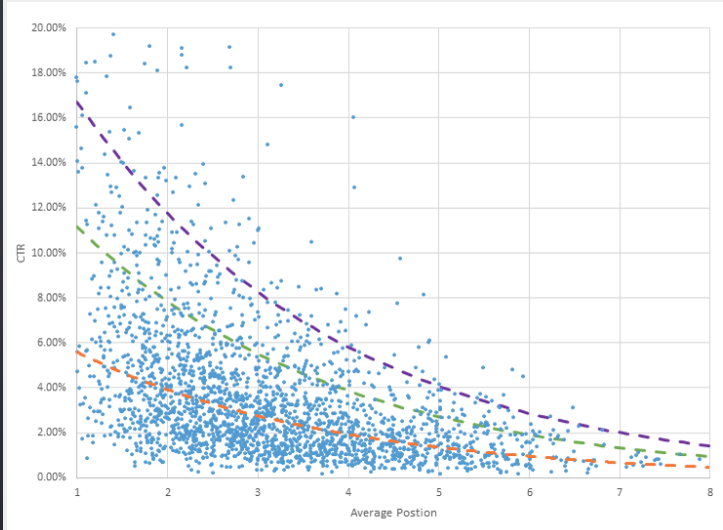## Linear regression

### Hypothesis

$h = \mathbf{θ}_0 + \mathbf{θ}_1 \times X$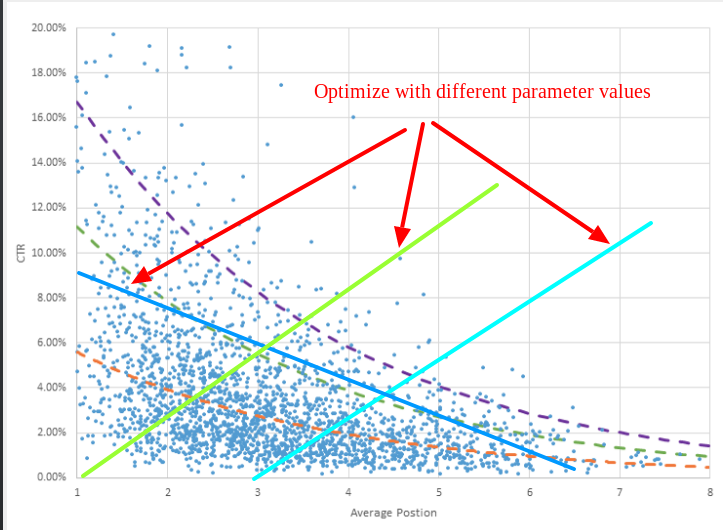• $Light green: \mathbf{θ}_0 = 0; \mathbf{θ}_1 = 0.1;$
• $Light blue: \mathbf{θ}_0 = -0.3; \mathbf{θ}_1 = 0.1;$
• $Dark blue: \mathbf{θ}_0 = 9; \mathbf{θ}_1 = -0.05;$

## Linear regression

### Hypothesis

$h = \mathbf{θ}_0 + \mathbf{θ}_1 \times X$

### Cost function

$J(θ) = \frac1{2m} \sum_{i=1}^m \left( h(X^{(i)}) - y^{(i)} \right)^2$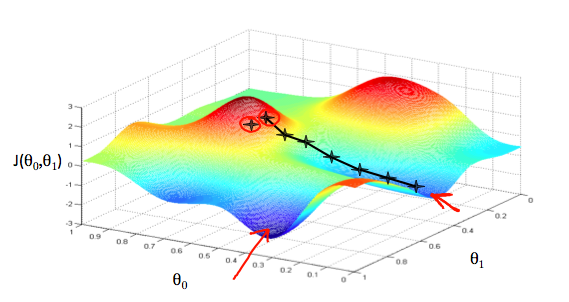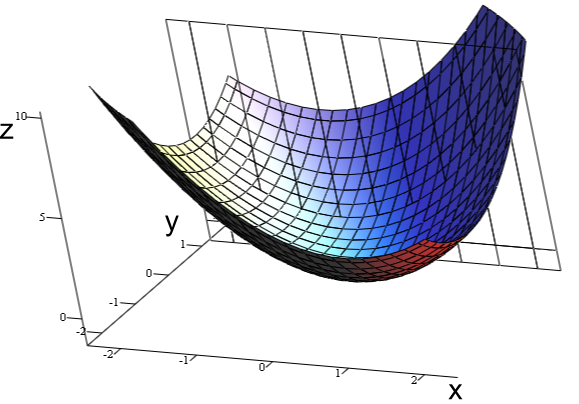By Indeed123 - commons, CC BY-SA 3.0, https://commons.wikimedia.org/w/index.php?curid=5508870

### Formal definition

$\mathbf{θ}_j = \mathbf{θ}_j - α \frac{\partial}{\partial\mathbf{θ}_j} J(θ)$
Repeat until convergence

## Gradient descent with one feature

### Derivatives

$\frac{\partial}{\partial\mathbf{θ}_0} J(θ) = \frac1m \sum_{i=1}^m \left( h(X^{(i)}) - y^{(i)} \right)$ $\frac{\partial}{\partial\mathbf{θ}_1} J(θ) = \frac1m \sum_{i=1}^m \left( h(X^{(i)}) - y^{(i)} \right) \times X^{(i)}$

## Linear regression

### Experimental dataYou can't bring the cost to 0!

## More features

$h = \mathbf{θ}_0 + \mathbf{θ}_1 \times {X}_1$
$h = \mathbf{θ}_0 + \mathbf{θ}_1 \times {X}_1 + \mathbf{θ}_2 \times {X}_2$
$h = \mathbf{θ}_0 + \mathbf{θ}_1 \times {X}_1 + ... \mathbf{θ}_N \times {X}_N$
Let's agree that ${X}_0 = 0$
$h = \mathbf{θ}_0 \times {X}_0 + \mathbf{θ}_1 \times {X}_1 + \mathbf{θ}_2 \times {X}_2 + ... \mathbf{θ}_N \times {X}_N$
$h = \mathbf{θ} \times {X}$

$\frac{\partial}{\partial\mathbf{θ}_0} J(θ) = \frac1m \sum_{i=1}^m \left( h(X^{(i)}) - y^{(i)} \right)$ $\frac{\partial}{\partial\mathbf{θ}_1} J(θ) = \frac1m \sum_{i=1}^m \left( h(X^{(i)}) - y^{(i)} \right) \times \mathbf{X}_1^{(i)}$ $\frac{\partial}{\partial\mathbf{θ}_2} J(θ) = \frac1m \sum_{i=1}^m \left( h(X^{(i)}) - y^{(i)} \right) \times \mathbf{X}_2^{(i)}$ ... $\frac{\partial}{\partial\mathbf{θ}_N} J(θ) = \frac1m \sum_{i=1}^m \left( h(X^{(i)}) - y^{(i)} \right) \times \mathbf{X}_N^{(i)}$

## Less features!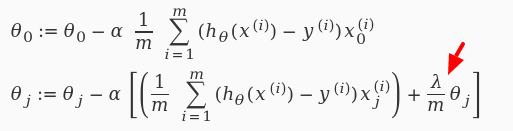## Validating the model

• Training set: 60%
• Cross validation set: 20%
• Test set: 20%

## Working with large data set$θ = θ - α \times \frac1{2m} \sum_{i=1}^m \left( h(X^{(i)}) - y^{(i)} \right) \times X$

## Working with large data set• Shuffle training set
• Perform gradient descent for single example
• Choose low α!

## Online learning

• Process training examples as they go
• Adjust parameters θ after every example
• Throw examples immediately
• Bonus: adjust to changing users preferences

## Decision making

• Visitor demographics (country/language)
• Time (weekday/hour)
• Previous history of actions
• Current page data (taxonomy)
• Update date of the content